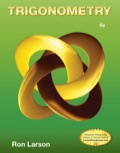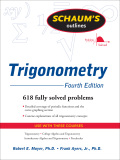TrigonometryHome   Algebra   Geometry   Arithmetic   Trigonometry   Probability/Statistics   Calculus   Mechanics GED   SAT   ACT   College Mathematics   GRE   School Supplies   About Us

Trigonometry

 Electronic Textbooks TDownloads - Preview, Get, Practice!Paperback Textbooks (New and Used)Examples
 Trigonometric Equations: Solve the equation, 2sin�θ - sinθ = 0                           This is a Quadratic Equation as in 2x� - x = 0                                          By factorization, Sinθ(2sinθ - 1) = 0                                                 So, sinθ = 0 and 2sinθ - 1 = 0                                                  When sinθ = 0, angle θ = 0�, 180� and 360�                                                        Also, for 2sinθ - 1 = 0                                                              sinθ = 0.5. Angle θ = 30� and 150�               Answer: Solutions of θ are 0�, 30�, 150�, 180� and 360 Polar Equations: Find the Polar Equation of the Circle whose Cartesian Equation is x� + y� = 4x.              If x = rcosθ and y = rsinθ         then r�cos�θ + r�sin�θ = 4rcosθ                 r�(cos�θ + sin�θ) = 4rcosθ                       Therefore, r� = 4rcosθ                                        r = 4cosθ                   Polar Equation is r = 4cosθfor Math Practice,

 More School Supplies and other useful items *  * *